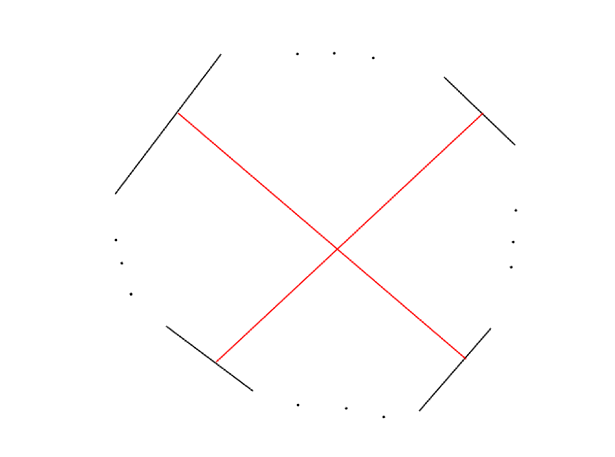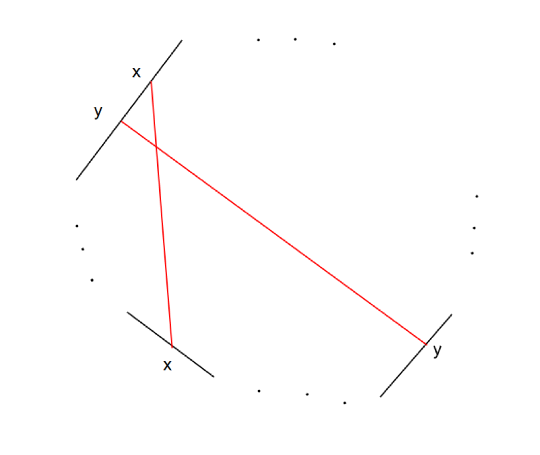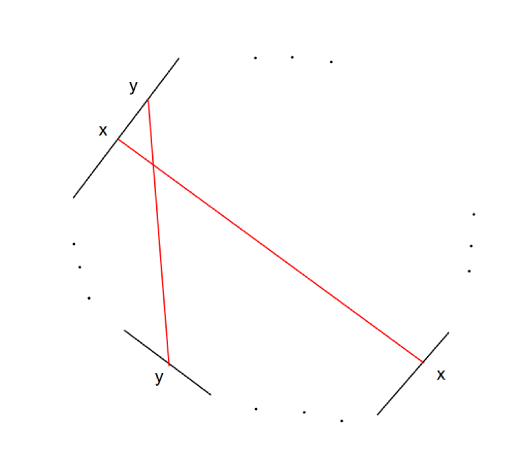# May 11, 2021 Single Round Match 804 Editorials

#### YetAnotherGraphColoring

Hint: Longest Decreasing Subsequence.

Fact 1: If for x, y x < y and a[x] > a[y] and for y, z y < z and a[y] > a[z] ⇒ x < z and a[x] > a[z].

Fact 2: Consider a sequence of indices i1, i2, i3, …, ik, where for each 1 < j ≤ k ij – 1 < ij and a[ij – 1] > a[ij]; then each pair of indices in the sequence are connected in our graph. So we need at least k colours.

What is the longest sequence satisfying the above conditions? Longest Decreasing Subsequence.

So if the length of LDS is k, we need at least k colours.

Read how to find the Longest Decreasing Subsequence here.

Just as when calculating LDS, let dpi be the length of longest decreasing sequence ending in i. For example for sequence 3, 2, 2, 1, 5, dp is [1, 2, 2, 3, 1].

If we assign colour dpi to i-th index, it’s proper colouring. Consider the opposite case, there exists x, y where x < y and a[x] > a[y] and dpx = dpy. As x < y and a[x] > a[y], we can add the y-th element to the LDS ending in x (with length dpx) and increase its length by 1, so dpy >= dpx + 1;  contradiction.

So the answer is the length of LDS. One can easily convert LDS to LIS by reversing the array.

Here is the code by at_f:

from collections import Counter, defaultdict, deque
from heapq import heapify, heappop, heappush
from bisect import bisect_left, bisect_right
import math
# import numpy as np

range = xrange

def lengthOfLIS(nums):
chain = [float("-inf")]
for x in nums:
newLen = bisect_left(chain, x)
assert chain[newLen - 1] < x
if newLen == len(chain):
chain.append(x)
else:
assert x <= chain[newLen]
chain[newLen] = x
return len(chain) - 1

class YetAnotherGraphColoring:
def minColors(self, n, a1, a2, x, y, z, m):
# Parameters: integer, integer, integer, integer, integer, integer, integer
# Returns: integer

a = [-1] * n
a = a1
a = a2
for i in range(2, n):
a[i] = (x * a[i - 1] + y * a[i - 2] + z) % m

lis = lengthOfLIS(a[::-1])
return lis



UnluckyNumber

Hint: Inclusion-exclusion.

We can count all of the possible options for assigning numbers, then subtract options where at least an edge is unlucky (i. e. sum of assigned numbers to its endpoints is z).

Let’s fix an edge to be unlucky, how many options are there for its endpoints? If we set the colour of one endpoint, the other has only one option. We can set one of the endpoints to max(1, z – k), 2, …, min(k, z – 1). So the number of options is min(k, z – 1) – max(1, z – k) + 1, let this number be len.

So we selected an edge and we want to calculate the number of colourings that this edge is unlucky in, the number of such colourings is k ^ (n – 2) * len. We have len options for endpoints of the corresponding edge and also k options for n – 2 remaining nodes.

This way, we subtracted some of invalid colourings twice. For example consider the case:

n = 4
m = 2
k = 1
z = 2
x[] = {1, 3}
y[] = {2, 4}

y[] = {2, 4}

The number of possible options must be 0, but with the above method we get -1.

We need to add the invalid colorings that counted twice and add them once. This results in a method known as inclusion-exclusion. Read about it here.

Let E be the set of all of the edges. Let f(A), where A is a subset of E, be the number of colorings where each edge in A is unlucky. The the answer isNow the remaining part is to calculate f(A). The vertices don’t have any edge in A have simply k options. Others will form several components using edges in A. It’s easy to understand that if we determine the colour of a vertex in a component, other vertices in the component will uniquely get colour. What if we have cycles? There is no problem with even cycles. But odd cycles sometimes make problems. If we set colour x for vertex a which lies in an odd cycle, x must be equal to z – x, so x = z / 2 and z needs to be even in this case.

To summarize, each component without an odd cycle has len options. Components with odd length and odd z have 0 options. Components with odd length and even z have 1 option.

To calculate f(A) we multiply options for single vertices and components.

Time complexity is O(2^m*m).

Code by heno239:

using namespace std;

//#define int long long
typedef long long ll;

typedef unsigned long long ul;
typedef unsigned int ui;
constexpr ll mod = 1000000007;
const ll INF = mod * mod;
typedef pair<int, int>P;
#define stop char nyaa;cin>>nyaa;
#define rep(i,n) for(int i=0;i<n;i++)
#define per(i,n) for(int i=n-1;i>=0;i--)
#define Rep(i,sta,n) for(int i=sta;i<n;i++)
#define rep1(i,n) for(int i=1;i<=n;i++)
#define per1(i,n) for(int i=n;i>=1;i--)
#define Rep1(i,sta,n) for(int i=sta;i<=n;i++)
#define all(v) (v).begin(),(v).end()
typedef pair<ll, ll> LP;
typedef long double ld;
typedef pair<ld, ld> LDP;
const ld eps = 1e-12;
const ld pi = acosl(-1.0);

ll mod_pow(ll x, ll n, ll m = mod) {
if (n < 0) {
ll res = mod_pow(x, -n, m);
return mod_pow(res, m - 2, m);
}
if (abs(x) >= m)x %= m;
if (x < 0)x += m;
ll res = 1;
while (n) {
if (n & 1)res = res * x % m;
x = x * x % m; n >>= 1;
}
return res;
}
struct modint {
ll n;
modint() :n(0) { ; }
modint(ll m) :n(m) {
if (n >= mod)n %= mod;
else if (n < 0)n = (n % mod + mod) % mod;
}
operator int() { return n; }
};
bool operator==(modint a, modint b) { return a.n == b.n; }
modint operator+=(modint& a, modint b) { a.n += b.n; if (a.n >= mod)a.n -= mod; return a; }
modint operator-=(modint& a, modint b) { a.n -= b.n; if (a.n < 0)a.n += mod; return a; }
modint operator*=(modint& a, modint b) { a.n = ((ll)a.n * b.n) % mod; return a; }
modint operator+(modint a, modint b) { return a += b; }
modint operator-(modint a, modint b) { return a -= b; }
modint operator*(modint a, modint b) { return a *= b; }
modint operator^(modint a, ll n) {
if (n == 0)return modint(1);
modint res = (a * a) ^ (n / 2);
if (n % 2)res = res * a;
return res;
}

ll inv(ll a, ll p) {
return (a == 1 ? 1 : (1 - p * inv(p % a, a)) / a + p);
}
modint operator/(modint a, modint b) { return a * modint(inv(b, mod)); }
modint operator/=(modint& a, modint b) { a = a / b; return a; }
const int max_n = 1 << 1;
modint fact[max_n], factinv[max_n];
void init_f() {
fact = modint(1);
for (int i = 0; i < max_n - 1; i++) {
fact[i + 1] = fact[i] * modint(i + 1);
}
factinv[max_n - 1] = modint(1) / fact[max_n - 1];
for (int i = max_n - 2; i >= 0; i--) {
factinv[i] = factinv[i + 1] * modint(i + 1);
}
}
modint comb(int a, int b) {
if (a < 0 || b < 0 || a < b)return 0;
return fact[a] * factinv[b] * factinv[a - b];
}
modint combP(int a, int b) {
if (a < 0 || b < 0 || a < b)return 0;
return fact[a] * factinv[a - b];
}

class UnluckyNumber {
public:
int numberOfColourings(int n, int m, int k, int z, vector <int> x, vector <int> y) {
vector<vector<int>> G(n);
rep(i, m) {
x[i]--; y[i]--;
}
int ri = min(z - 1, k);
int le = max(1, z - k);
modint len = ri - le + 1;
vector<bool> used(n);
vector<int> col(n);
modint ans = 0;
rep(i, (1 << m)) {
rep(j, n)G[j].clear();
fill(all(used), false);
rep(j, m) {
if (i & (1 << j)) {
G[x[j]].push_back(y[j]);
G[y[j]].push_back(x[j]);
}
}
modint num = 1;
rep(j, n) {
if (used[j])continue;
int cnt = 1; used[j] = true;
queue<int> q; q.push(j);
bool isb = true;
col[j] = 0;
while (!q.empty()) {
int id = q.front(); q.pop();
for (int to : G[id]){
if (!used[to]) {
cnt++;
used[to] = true;
col[to] = col[id] ^ 1;
q.push(to);
}
else {
if (col[to] != (col[id] ^ 1))isb = false;
}
}
}
if (cnt == 1)num *= k;
else {
if (isb) {
num *= len;
}
else {
if (z % 2 == 0)num *= 1;
else num *= 0;
}
}
}
int coef = 1;
rep(j, m)if (i & (1 << j))coef *= -1;
ans += (modint)coef * num;
}
return ans;
}
};



#### PolygonAndPermutations

Hint: Count inversions.

There are some intersections that it’s impossible to avoid. Consider the intersection between two segments (a, b), (b, d). If a, b, c, d belong to 3 or 4 different line segments, it’s impossible to avoid this intersection.

When a, b, c, d belong to 4 different line segments:It’s not depend on the order we put the line segments or direction of segments (upside down or not), we’ll always have this intersection.

When a, b, c, d belong to 3 different line segments:One may wonder if we make the top line segment upside down this intersection will resolve. But We’ll then have this intersection again:So the only intersections we can minimize are those that a, b, c, d belong to two segments. So the order we put segments is not important and the only point is which segments we’re using “upside down”. For each two segments, we’ll calculate the number of intersections if we use them (1) both normally or both upside down and (2) one normally and one upside down.

Let’s calculate for two segments (with fixed direction) how many intersections are there. Calculation is easy in O(n^2); although one can calculate it in O(n log n).

At last we bruteforce on the direction of segments. Which segments we’ll use in upside down mode and which normal mode. Then we’ll calculate the number of intersections using the previously preprocessed values.

Code by tourist:

class PolygonAndPermutations {
public:
long long solve(int, int, long long);
};

long long xx, pr;

long long get_random() {
xx = xx % 1000000007;
long long temp = (xx*xx + pr) % 1000000007;
pr = xx;
xx = temp;
return xx;
}

vector<int> permutation(int n) {
vector<int> cur(n), res;
for(int i=0; i<n; ++i) cur[i] = i+1;
for(int i=0; i<n; ++i) {
int tmp = get_random() % (n-i);
swap( cur[n-i-1], cur[tmp] );
res.push_back(cur[n-i-1]);
}
return res;
}

long long PolygonAndPermutations::solve(int K, int N, long long seed) {
xx = seed;
pr = 0;
vector< vector<int> > segments(K);
for (int i=0; i<K; ++i) {
segments[i] = permutation(N);
assert((int) segments[i].size() == N);
for (int j = 0; j < N; j++) segments[i][j] -= 1;
}
for (int i = 0; i < K; i++) {
for (int j = i + 1; j < K; j++) {
for (int i2 = 0; i2 < K; i2++) {
for (int j2 = i2 + 1; j2 < K; j2++) {
if (make_pair(i, j) < make_pair(i2, j2)) {
if (i != i2 && i != j2 && j != i2 && j != j2) {
if (i < i2 && i2 < j && j < j2) {
}
continue;
}
assert(i == i2 || i == j2 || j == i2 || j == j2);
add += N * (N - 1) / 2;
}
}
}
}
}
vector<vector<int>> mat(K, vector<int>(K));
for (int i = 0; i < K; i++) {
for (int j = i + 1; j < K; j++) {
vector<int> pos_j(N);
for (int t = 0; t < N; t++) {
pos_j[segments[j][t]] = t;
}
for (int t0 = 0; t0 < N; t0++) {
for (int t1 = t0 + 1; t1 < N; t1++) {
if (pos_j[segments[i][t0]] < pos_j[segments[i][t1]]) {
mat[i][j] -= 1;
} else {
mat[i][j] += 1;
}
}
}
}
}
long long ans = (long long) 1e18;
for (int t = 0; t < (1 << K); t++) {
long long cur = 0;
for (int i = 0; i < K; i++) {
for (int j = i + 1; j < K; j++) {
if ((t >> i) % 2 != (t >> j) % 2) {
cur += mat[i][j];
}
}
}
ans = min(ans, cur);
}
}



#### CostMaximizer

Hint: I wish it was possible to select all vertices from the same color.

Consider we select a set of vertices S from blue and others from red. Then answer isSo we want to minimizeSpend O(n^2) and fix v, u such that v is a vertex with red color and u is a vertex from blue colour (we know that these vertices exists).

So we want to put vertices in two groups, one with v and one with u, such that the sum of weights of edges (2 * w – k) connecting vertices from different groups becomes minimum. This is the definition of minimum cut which is equal to maximum flow. Using max-flow we can solve the problem.

Code by fetetriste:

public class CostMaximizer {
final int infinity = (int) 1e9;

public long maxPossible(int n, int K, int[] inputW) {
int ptr = 0;
int[][] w = new int[n][n];
long sum = 0;
for (int i = 0; i < n; i++) {
for (int j = i + 1; j < n; j++) {
w[i][j] = inputW[ptr];
w[j][i] = inputW[ptr];
++ptr;
sum += w[i][j];
}
}

long min = infinity;
for (int s = 0; s < n; s++) {
for (int t = s + 1; t < n; t++) {
Graph g = new Graph(n + 2);
for (int i = 0; i < n; i++) {
for (int j = i + 1; j < n; j++) {
g.addEdge(i, j, 2 * w[i][j] - K);
g.addEdge(j, i, 2 * w[i][j] - K);
}
}
min = Math.min(min, g.minCut());
}
}

return sum - min;
}

class Graph {
int n;
int s;
int t;
int[] firstEdge;
int[] nextEdge;
int[] edgeDst;
int[] edgeCap;
int[] edgeFlow;
int numEdges;
int[] level;
int[] q;
int[] lastEdge;

Graph(int n) {
this.n = n;
s = n - 1;
t = n - 2;
firstEdge = new int[n];
final int initialEdges = 10;
nextEdge = new int[initialEdges];
edgeDst = new int[initialEdges];
edgeCap = new int[initialEdges];
edgeFlow = new int[initialEdges];
numEdges = 0;
level = new int[n];
q = new int[n];
lastEdge = new int[n];
Arrays.fill(firstEdge, -1);
}

void reallocate() {
int k = nextEdge.length * 3 / 2 + 1;
nextEdge = Arrays.copyOf(nextEdge, k);
edgeDst = Arrays.copyOf(edgeDst, k);
edgeCap = Arrays.copyOf(edgeCap, k);
edgeFlow = Arrays.copyOf(edgeFlow, k);
}

void addEdge(int a, int b, int cap) {
if (numEdges + 2 >= nextEdge.length) {
reallocate();
}
int e = numEdges++;
nextEdge[e] = firstEdge[a];
firstEdge[a] = e;
edgeDst[e] = b;
edgeCap[e] = cap;
edgeFlow[e] = 0;

e = numEdges++;
nextEdge[e] = firstEdge[b];
firstEdge[b] = e;
edgeDst[e] = a;
edgeCap[e] = 0;
edgeFlow[e] = 0;
}

long minCut() {
return maxFlow();
}

long maxFlow() {
long flow = 0;
while (bfs()) {
for (int i = 0; i < n; i++) {
lastEdge[i] = firstEdge[i];
}
while (true) {
break;
}
}
}
return flow;
}

int dfs(int v, int delta) {
if (v == t) {
return delta;
}
for (; lastEdge[v] >= 0; lastEdge[v] = nextEdge[lastEdge[v]]) {
int e = lastEdge[v];
int u = edgeDst[e];
if (level[u] != level[v] + 1) {
continue;
}
int x = Math.min(delta, edgeCap[e] - edgeFlow[e]);
if (x <= 0) {
continue;
}
x = dfs(u, x);
if (x > 0) {
edgeFlow[e] += x;
edgeFlow[e ^ 1] -= x;
return x;
}
}
level[v] = -1;
return 0;
}

boolean bfs() {
Arrays.fill(level, -1);
q = s;
level[s] = 0;
int qt = 0;
int qh = 1;
while (qt < qh) {
int v = q[qt++];
for (int e = firstEdge[v]; e >= 0; e = nextEdge[e]) {
int u = edgeDst[e];
if (edgeFlow[e] < edgeCap[e] && level[u] < 0) {
level[u] = level[v] + 1;
q[qh++] = u;
}
}
}
return level[t] >= 0;
}

}

}


a.poorakhavan

Guest Blogger

categories & Tags

UNLEASH THE GIG ECONOMY. START A PROJECT OR TALK TO SALES
Close